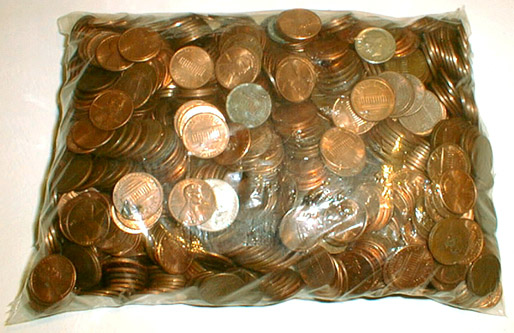to english | index | next

1184枚の硬貨から成る12米ドル10セント (金額第二七番)
US\$12.10 Which Consists of 1184 Coins (Money Amount No. 27)

The U. S. coins, a plastic bag
2002

cf. 1184 and 1210 are the second smallest amicable pair which Paganini found in 1866.
(Two numbers are called amicable if each equals to the sum of the divisors of the other.)
Divisors of 1184: 1 + 2 + 4 + 8 + 16 + 32 + 37 + 74 + 148 + 296 + 592 = 1210
Divisors of 1210: 1 + 2 + 5 + 10 + 11 + 22 + 55 + 110 + 121 + 242 + 605 = 1184